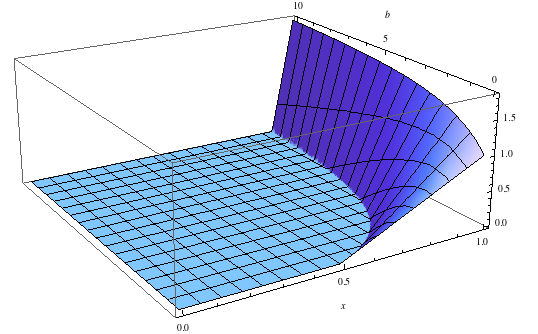# Minimal continuous poker

Consider the following poker-like game, played with two players: Alice and Bob.  Bob posts a blind of 1.  Both players are dealt a single, continuous hand chosen uniformly at random from $[0,1]$.  Alice can fold, call, or raise any amount $b \gt 0$ (calling means $b = 0$).  Bob either calls or folds.

My original plan was to work out the Nash equilibrium for this game, and therefore derive interesting smooth curves describing the optimal way for Alice to bluff and generally obscure her hand.  It didn’t quite work out that way, but the result is still interesting.

Let $A$ be the optimal expected payoff for Alice, $A(x)$ the optimal expected payoff given Alice’s specific hand x.  Clearly A(x) is a weakly increasing function of x; if x’ > x but A(x’) < A(x), Alice can improve A(x’) to A(x) by pretending she has hand x and using the same strategy.  If A(x) > 0 for some x, so that Alice’s optimal strategy is better than folding, then Alice will never fold since any nonzero probability of folding would reduce her utility.  Thus there is a threshold hand $x_t$ below which Alice always folds (technically, always “might as well” fold, but we’ll gloss over this detail for the moment), and above which Alice always calls but might vary the amount she raises by.  A similar argument applied to Bob gives a threshold function $y_t(b)$ s.t. Bob folds if $y < y_t(b)$ and calls if $y > y_t(b)$.

These and other similar preliminaries aside, I spent a while banging my head against the wall before temporarily giving up and picking a simpler game.  Specifically, make the bet $b$ a fixed constant.  If $b = 0$, Bob always checks, and Alice’s optimal strategy is (unsurprisingly) to call if $x > 1/2$.  This gives a payoff of $A = 1/4$.  If $b > 0$, Bob’s function $y_t(b)$ is a constant. The values of $x_t$, $y_t$, and $A$ at the Nash equilibrium turn out to be

\begin{aligned} x_t =~& \frac{2+2b+b^2}{(2+b)^2} \\\ y_t =~& \frac{1+b}{2+b} \\\ A =~& \frac{2+2b}{(2+b)^3} \end{aligned}

A few notes: for any $b > 0$, $x_t < y_t$, since Bob knows Alice’s threshold and gains nothing by calling with hands only slightly over $x_t$.  Interestingly, Alice’s payoff remains unchanged if she varies her threshold anywhere satisfying $x_t < y_t$; the particular value of $x_t$ is only prevents Bob from increasing his utility with a different value of $y_t$.  However, the most important property of this result is that $A$ is a decreasing function of $b$.  If the bet is fixed, Alice would prefer it to be zero so that $A = 1/4$.

Now back the general game, where Alice chooses the bet.  We have $A \ge 1/4$, since Alice can choose to always call or fold.  Can she do better, varying the bet in some exotic fashion so as to milk more money out of Bob while keeping her hand disguised?  Well, no.  Update: After disproving a conjecture (see below), the answer is maybe.

Let’s see what happens if Bob follows the optimal strategy from the fixed $b$ game, calling if $y > (1+b)/(2+b)$ and folding otherwise. Since Bob’s strategy is fixed, Alice can maximize her utility without any extra randomness, and we need only consider bets which are a deterministic function of $x$.  For $x < x_t$ she folds and $A(x) = 0$, for $x > x_t$ she bets $b = b(x)$ with payoff

\begin{aligned} A(x) =~& 1 Pr(y_t > y) + (1+b) Pr(x > y > y_t) - (1+b) Pr(y > x,y_t) \\\ =~& y_t + (1+b) \max(0,x-y_t) - (1+b) (1 - \max(x,y_t)) \\\ =~& y_t + (1+b) \max(0,x-y_t) - (1+b) + (1+b)x + (1+b) \max(0,y_t-x) \\\ =~& y_t + (1+b)(x-1) + (1+b) |x-y_t| \\\ \end{aligned}

Differentiating w.r.t. $b$,

\begin{aligned} A(x)’ =~& x - 1 + y_t’ + |x-y_t| + (1+b) sgn(y_t - x) y_t’ \\\ y_t’ =~& \frac{1}{2+b} - \frac{1+b}{(2+b)^2} = \frac{1 - y_t}{2+b} \end{aligned}

If $x < y_t$, this becomes

\begin{aligned} A(x)' =~& x - 1 + y_t’ + y_t - x + (1+b) y_t’ \\\ =~& -1 + (2 + b) (1 - y_t) / (2+b) + y_t \\\ =~& -1 + 1 - y_t + y_t = 0 \end{aligned}

so if $x < y_t$, Alice’s bet doesn’t matter.  If $x > y_t$, we have

\begin{aligned} A(x)’ =~& x - 1 + y_t’ + x-y_t - (1+b) y_t’ \\\ =~& 2x-1 - b y_t’ - y_t \\\ =~& 2x-1 - b (1 - y_t)/(2+b) - y_t \\\ =~& 2x-1 - b/(2+b) + b(1+b)/(2+b)^2 - (1+b)/(2+b) \\\ =~& 2x-1 - \frac{2b+b^2 - b - b^2 + 2 + 3b + b^2}{(2+b)^2} \\\ =~& 2x-1 - \frac{2 + 4b + b^2}{(2+b)^2} \\\ =~& 2x-2 + \frac{2}{(2+b)^2} \\\ \end{aligned}

which is negative as $b \to \infty$ and zero at $b = \sqrt{1/(1-x)-2}$.

Thus $A(x)’ = 0$ iff $(2+b)^2 = 1/(1-x)$, or

$$b = \sqrt{\frac{1}{1-x} - 2}$$

Unfortunately for Bob, $A(x)’ > 0$ at $b = 0$ iff $2x-2+1/2 > 0$ iff $x > 3/4$.  Thus Alice can beat $A = 1/4$ by increasing her bet whenever $x > 3/4$.  Abandoning hand calculation and turning to Mathematica, it turns out Alice’s optimal strategy achieves $A = 7/24 \approx .29$.

So, we haven’t found the Nash equilibrium yet, but at least our minimal poker game isn’t (necessarily) incredibly boring.  More analysis is required.  For pretty pictures’ sake, here’s a plot of Alice’s outcome as $x$ and $b$ vary: